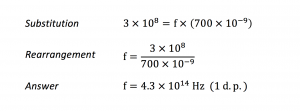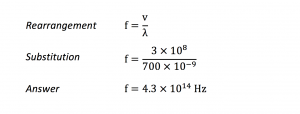Physics 16/10/2018

# Equations in GCSE Physics

By KEVIN QUINN

Yes, it’s true, there’s a lot of maths in physics. If you take a look at the physics equation sheet on My GCSE Science, you’ll see that for the new 9-1 GCSE there are over twenty equations which you’ll have to remember by the end of Year 11! This blog helps you make sense of it all.

## The memory game

Take a look at the physics equation sheet at the top of the video dashboard on My GCSE Science. On top of this long list, the exam board will provide you with a few extra equations on a formula page within your GCSE Physics papers.

The sheer number of equations in GCSE Physics can be off-putting at first. But as you work through the course, watch My GCSE Science videos and tackle as many exam-style questions as possible, most of them will naturally burrow their way into your memory. That’s the best – and easiest – way to learn equations. For a few equations that don’t stick in your memory, and aren’t provided on the formula sheet, you’ll just have to learn them off by heart, the old-school way.

## Practice makes perfect

Remember that, on top of memorising the equations, you’ll also have to understand how to use them. That means practice.

When using the My GCSE Science physics videos, press ‘pause’ each time a new example appears on the screen. Have a go at the question before checking whether your answer matches the one that appears on the video. After watching the video, take a quick break before trying the exam-style questions. You’ll know you’ve got it when you can complete the maths-heavy questions without referring back to the video or the equation sheet.

## Work it

You’ve already heard this a thousand times, but here goes again: always show your working (and the correct unit, if required). If your working is correct but you’ve fallen at the final hurdle and written the wrong answer, you might get away with losing just one mark. If you haven’t shown any working and your answer is wrong, you’ll get no marks at all!

## Substitution and rearrangement

To substitute then rearrange, or to rearrange then substitute? To help explain the difference between these two methods of working with equations, let’s look at this short mathematical question.

EXAMPLE.  Calculate the frequency of red light of wavelength 700 nm.  The speed of light in vacuum is 3 × 108 m/s.

#### Method 1: substituting then rearranging

The correct equation to use here is v=f λ. If we remember that 1 nm = 10–9 m, we can calculate the final answer as follows:#### Method 2: rearranging then substituting

This time, we are going to rearrange  to make f the subject before substituting the values from the question. Remember that to make f the subject in this equation, we divide both sides of the equation by  λ.Both methods are very similar – it’s just that the order of the steps is different. Method 2 is more common at A-level as it helps you avoid mixing up numbers in more complicated equations. But at GCSE, just stick with the method that works best for you. Good luck!

Kevin Quinn